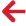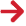﻿ PipeLay > Excel Add-In > Function Reference > Statistics Functions > PLGetEnvelopeData

# PLGetEnvelopeData

Navigation:  PipeLay > Excel Add-In > Function Reference > Statistics Functions >

# PLGetEnvelopeData## PURPOSE

Retrieves both the maximum and minimum values necessary to create an envelope plot. For Bending Stress, the minimum value is different than that returned by PLGetMininmumValue as it is calculated differently. (Bending Stress returns the maximum value always so the PLGetMinimumValue returns the minimum of that Maximum value)

Note: Adding this formula to Excel is also different. First, highlight 2 adjacent cells on the same row, such as columns A and B on row 1. Enter the formula as desired but instead of just pressing ENTER, press CTRL+SHIFT+ENTER. The formula will them be shown with curly braces around it when the cell is highlighted and 2 values will be returned from the function.

## SYNTAX

PLGetEnvelopeData( DatabaseName, StartTimeIndex, EndTimeIndex, ParameterType, ItemIndex, DOFNo )

## PARAMETERS

 DatabaseName (Character String) The full path and filename to the relevant database. The file extension is optional. StartTimeIndex (Integer) The start time index, from 1 to the number of solutions in the database. EndTimeIndex (Integer) The end time index, from 1 to the number of solutions in the database. This should be a number higher than StartTimeIndex. ParameterType (Integer) The type of parameter to retrieve data for. See Parameter Types for a complete list. ItemIndex (Integer) The index of the required item. This is parameter dependent e.g. for kinematic parameters, this is a node number. DOFNo (Integer) The DOF or Local node number. This is parameter dependent so the ranges should correspond to what is valid for the parameter.

## OUTPUT

A pair of numbers, or the first number if entered as a normal formula, containing the maximum and minimum values for the specified parameters or one of the following error codes:

 -999999998 Invalid Database. -999999996 Invalid Parameter Number. -999999995 Invalid Time Index. This may be either the start or end time. -999999990 No Data Available. -999999988 Item Index is out of range. -999999985 Local Node Number or DOF is out of range. -999999980 General Read Error.

Error Codes

Parameters

PLGetMaximumValue

PLGetMeanValue

PLGetMedian

PLGetMode

PLGetMinimumValue

PLGetNaiveStandardDeviation

PLGetRangeValue

PLGetStandardDeviation